# MapReduce Program – Finding The Average Age of Male and Female Died in Titanic Disaster

• Difficulty Level : Medium
• Last Updated : 08 Oct, 2021

All of us are familiar with the disaster that happened on April 14, 1912. The big giant ship of 46000-ton in weight got sink-down to the depth of 13,000 feet in the North Atlantic Ocean. Our aim is to analyze the data obtained after this disaster. Hadoop MapReduce can be utilized to deal with this large datasets efficiently to find any solution for a particular problem.

Problem Statement: Analyzing the Titanic Disaster dataset, for finding the average age of male and female persons died in this disaster with MapReduce Hadoop.

### Step 1:

We can download the Titanic Dataset from this Link. Below is the column structure of our Titanic dataset. It consists of 12 columns where each row describes the information of a particular person.### Step 2:

The first 10 records of the dataset is shown below.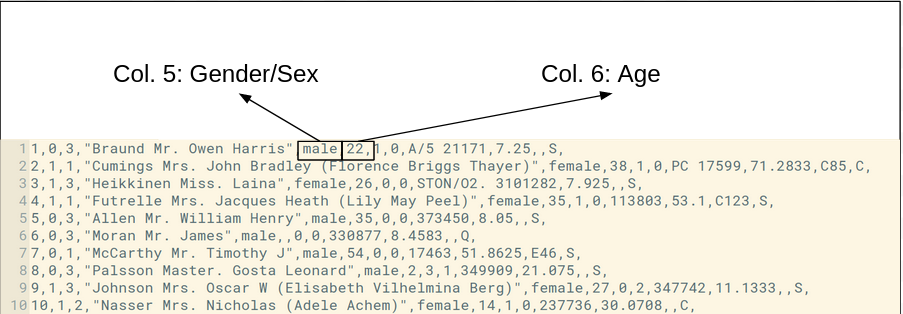### Step 3:

Make the project in Eclipse with below steps:

• First Open Eclipse -> then select File -> New -> Java Project ->Name it Titanic_Data_Analysis -> then select use an execution environment -> choose JavaSE-1.8 then next -> Finish.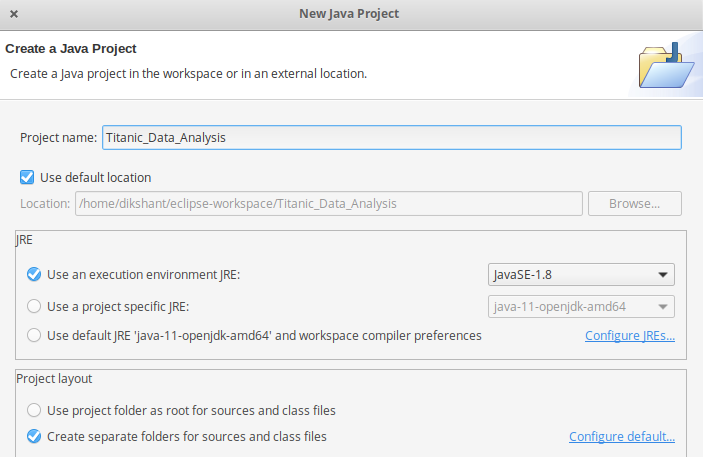• In this Project Create Java class with name Average_age -> then click Finish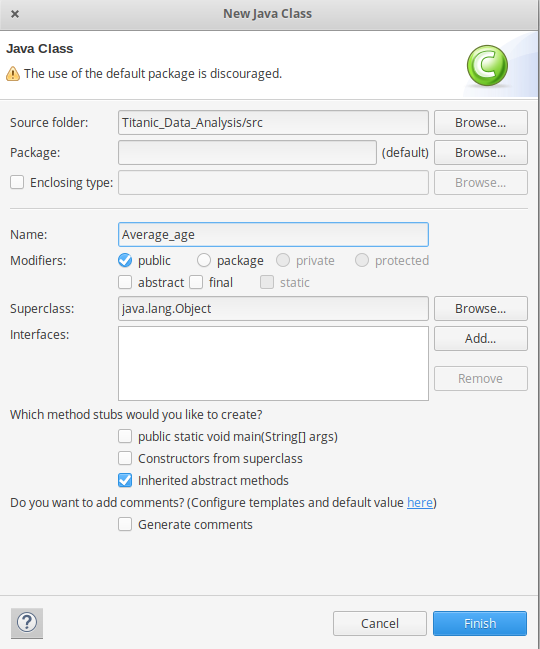• Copy the below source code to this Average_age java class

## Java

 `// import libraries``import` `java.io.IOException;``import` `org.apache.hadoop.fs.Path;``import` `org.apache.hadoop.conf.*;``import` `org.apache.hadoop.io.*;``import` `org.apache.hadoop.mapreduce.*;``import` `org.apache.hadoop.mapreduce.lib.input.FileInputFormat;``import` `org.apache.hadoop.mapreduce.lib.input.TextInputFormat;``import` `org.apache.hadoop.mapreduce.lib.output.FileOutputFormat;``import` `org.apache.hadoop.mapreduce.lib.output.TextOutputFormat;`` ` `// Making a class with name Average_age``public` `class` `Average_age {`` ` `    ``public` `static` `class` `Map ``extends` `Mapper {`` ` `        ``// private text gender variable which``        ``// stores the gender of the person``        ``// who died in the Titanic Disaster``        ``private` `Text gender = ``new` `Text();`` ` `        ``// private IntWritable variable age will store``        ``// the age of the person for MapReduce. where``        ``// key is gender and value is age``        ``private` `IntWritable age = ``new` `IntWritable();`` ` `        ``// overriding map method(run for one time for each record in dataset)``        ``public` `void` `map(LongWritable key, Text value, Context context) ``throws` `IOException, InterruptedException``        ``{`` ` `            ``// storing the complete record``            ``// in a variable name line``            ``String line = value.toString();`` ` `            ``// splitting the line with ', ' as the``            ``// values are separated with this``            ``// delimiter``            ``String str[] = line.split(``", "``);`` ` `            ``/* checking for the condition where the``               ``number of columns in our dataset``               ``has to be more than 6. This helps in``               ``eliminating the ArrayIndexOutOfBoundsException``               ``when the data sometimes is incorrect``               ``in our dataset*/``            ``if` `(str.length > ``6``) {`` ` `                ``// storing the gender``                ``// which is in 5th column``                ``gender.set(str[``4``]);`` ` `                ``// checking the 2nd column value in``                ``// our dataset, if the person is``                ``// died then proceed.``                ``if` `((str[``1``].equals(``"0"``))) {`` ` `                    ``// checking for numeric data with``                    ``// the regular expression in this column``                    ``if` `(str[``5``].matches(``"\\d+"``)) {`` ` `                        ``// converting the numeric``                        ``// data to INT by typecasting``                        ``int` `i = Integer.parseInt(str[``5``]);`` ` `                        ``// storing the person of age``                        ``age.set(i);``                    ``}``                ``}``            ``}``            ``// writing key and value to the context``            ``// which will be output of our map phase``            ``context.write(gender, age);``        ``}``    ``}`` ` `    ``public` `static` `class` `Reduce ``extends` `Reducer {`` ` `        ``// overriding reduce method(runs each time for every key )``        ``public` `void` `reduce(Text key, Iterable values, Context context)``            ``throws` `IOException, InterruptedException``        ``{`` ` `            ``// declaring the variable sum which``            ``// will store the sum of ages of people``            ``int` `sum = ``0``;`` ` `            ``// Variable l keeps incrementing for``            ``// all the value of that key.``            ``int` `l = ``0``;`` ` `            ``// foreach loop``            ``for` `(IntWritable val : values) {``                ``l += ``1``;``                ``// storing and calculating``                ``// sum of values``                ``sum += val.get();``            ``}``            ``sum = sum / l;``            ``context.write(key, ``new` `IntWritable(sum));``        ``}``    ``}`` ` `    ``public` `static` `void` `main(String[] args) ``throws` `Exception``    ``{``        ``Configuration conf = ``new` `Configuration();`` ` `        ``@SuppressWarnings``(``"deprecation"``)``        ``Job job = ``new` `Job(conf, ``"Averageage_survived"``);``        ``job.setJarByClass(Average_age.``class``);`` ` `        ``job.setMapOutputKeyClass(Text.``class``);``        ``job.setMapOutputValueClass(IntWritable.``class``);``        ` `        ``// job.setNumReduceTasks(0);``        ``job.setOutputKeyClass(Text.``class``);``        ``job.setOutputValueClass(IntWritable.``class``);`` ` `        ``job.setMapperClass(Map.``class``);``        ``job.setReducerClass(Reduce.``class``);`` ` `        ``job.setInputFormatClass(TextInputFormat.``class``);``        ``job.setOutputFormatClass(TextOutputFormat.``class``);`` ` `        ``FileInputFormat.addInputPath(job, ``new` `Path(args[``0``]));``        ``FileOutputFormat.setOutputPath(job, ``new` `Path(args[``1``]));``        ``Path out = ``new` `Path(args[``1``]);``        ``out.getFileSystem(conf).delete(out);``        ``job.waitForCompletion(``true``);``    ``}``}`
`hadoop version`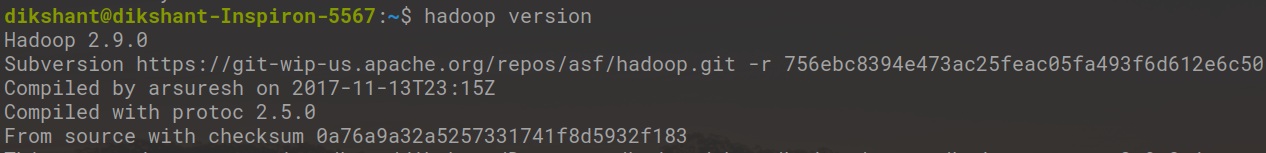• Now we add these external jars to our Titanic_Data_Analysis project. Right Click on Titanic_Data_Analysis -> then select Build Path-> Click on Configure Build Path and select Add External jars…. and add jars from it’s download location then click -> Apply and Close.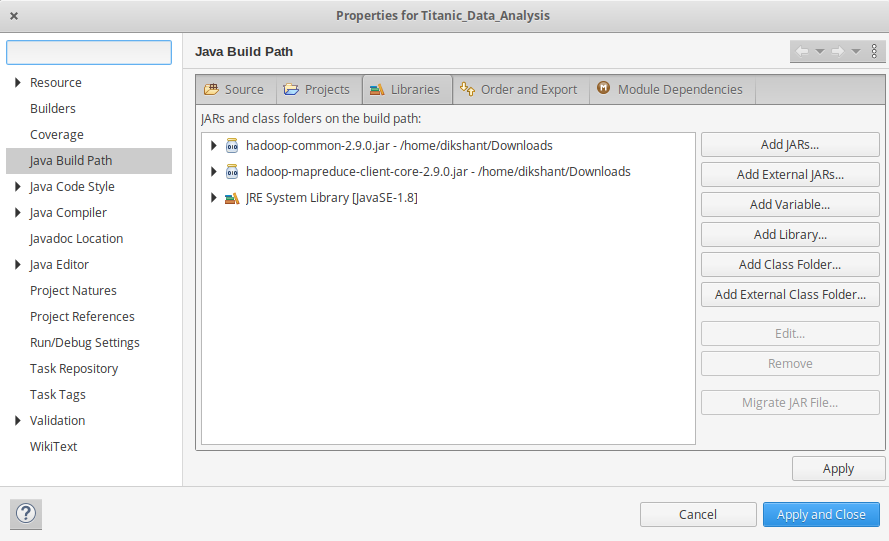• Now export the project as jar file. Right-click on Titanic_Data_Analysis choose Export.. and go to Java -> JAR file click -> Next and choose your export destination then click -> Next. Choose Main Class as Average_age by clicking -> Browse and then click -> Finish -> Ok.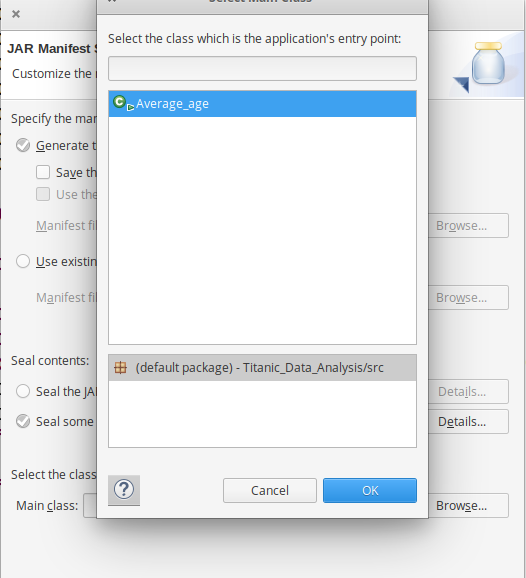### Step 4:

`start-dfs.sh `
`start-yarn.sh`

`jps `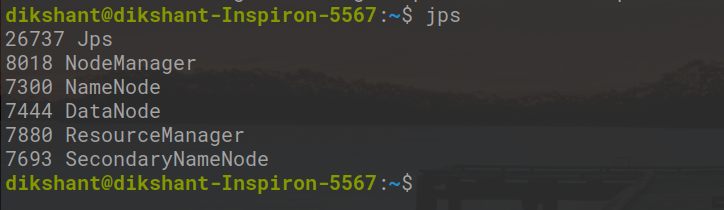### Step 5:

Syntax:

`hdfs dfs -put /file_path /destination`

In below command / shows the root directory of our HDFS.

`hdfs dfs -put /home/dikshant/Documents/titanic_data.txt /`

Check the file sent to our HDFS.

`hdfs dfs -ls /`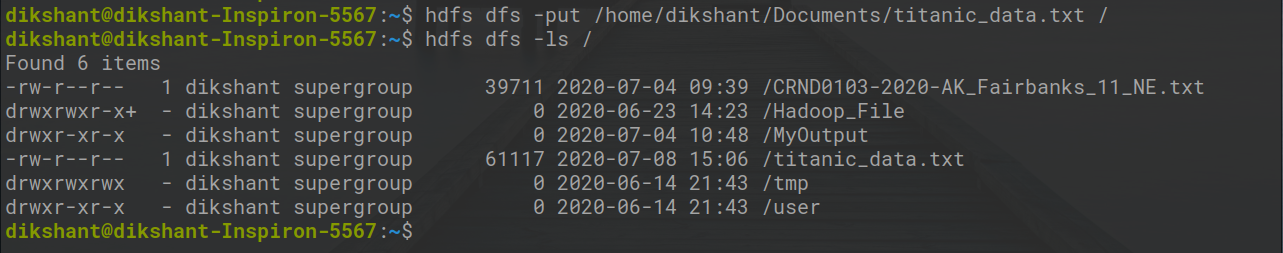### Step 6:

Now Run your Jar File with below command and produce the output in Titanic_Output File.

Syntax:

`hadoop jar /jar_file_location /dataset_location_in_HDFS /output-file_name`

Command:

`hadoop jar /home/dikshant/Documents/Average_age.jar /titanic_data.txt /Titanic_Output `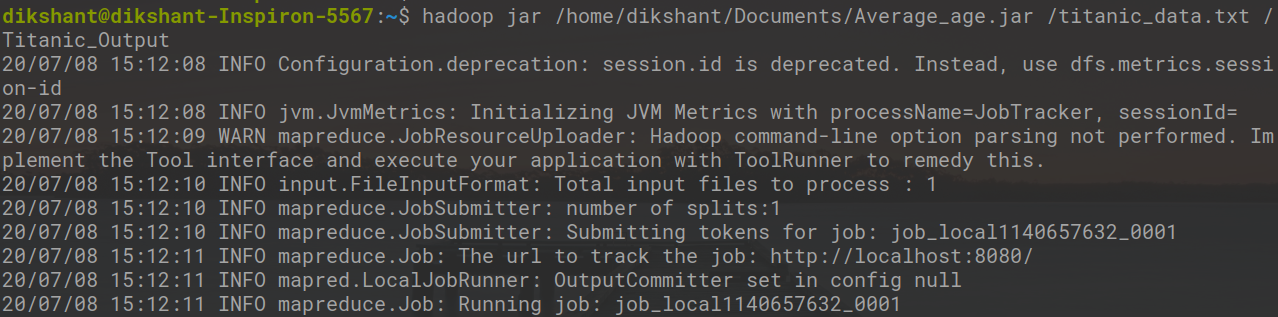### Step 7:

Now Move to localhost:50070/, under utilities select Browse the file system and download part-r-00000 in /MyOutput directory to see result.

Note: We can also view the result with below command

`hdfs dfs -cat /Titanic_Output/part-r-00000 `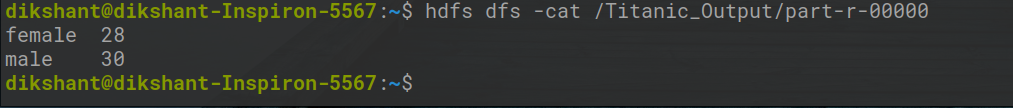In the above image, we can see that the average age of the female is 28 and male is 30 according to our dataset who died in the Titanic Disaster.

My Personal Notes arrow_drop_up